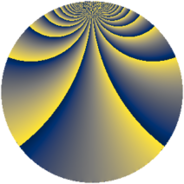# Properties

 Label 228.2.mLevel $228$ Weight $2$ Character orbit 228.m Rep. character $\chi_{228}(11,\cdot)$ Character field $\Q(\zeta_{6})$ Dimension $72$ Newform subspaces $1$ Sturm bound $80$ Trace bound $0$

# Related objects

## Defining parameters

 Level: $$N$$ $$=$$ $$228 = 2^{2} \cdot 3 \cdot 19$$ Weight: $$k$$ $$=$$ $$2$$ Character orbit: $$[\chi]$$ $$=$$ 228.m (of order $$6$$ and degree $$2$$) Character conductor: $$\operatorname{cond}(\chi)$$ $$=$$ $$228$$ Character field: $$\Q(\zeta_{6})$$ Newform subspaces: $$1$$ Sturm bound: $$80$$ Trace bound: $$0$$

## Dimensions

The following table gives the dimensions of various subspaces of $$M_{2}(228, [\chi])$$.

Total New Old
Modular forms 88 88 0
Cusp forms 72 72 0
Eisenstein series 16 16 0

## Trace form

 $$72q + 3q^{6} + O(q^{10})$$ $$72q + 3q^{6} - 8q^{10} - 10q^{12} - 4q^{16} - 22q^{18} + 4q^{21} - 6q^{22} + 11q^{24} + 16q^{25} + 6q^{28} - 60q^{30} - 30q^{33} - 20q^{34} - 11q^{36} - 8q^{37} + 36q^{40} + 8q^{42} - 28q^{45} - 8q^{46} - 23q^{48} - 16q^{49} + 6q^{52} - 22q^{54} - 6q^{57} - 80q^{58} - 14q^{60} - 40q^{61} + 48q^{64} - 15q^{66} + 4q^{69} + 72q^{70} + 15q^{72} - 4q^{73} + 12q^{76} + 44q^{78} + 4q^{81} - 6q^{82} + 56q^{84} - 52q^{85} - 44q^{88} + 20q^{90} + 4q^{93} + 56q^{94} - 86q^{96} - 16q^{97} + O(q^{100})$$

## Decomposition of $$S_{2}^{\mathrm{new}}(228, [\chi])$$ into newform subspaces

Label Dim. $$A$$ Field CM Traces $q$-expansion
$$a_2$$ $$a_3$$ $$a_5$$ $$a_7$$
228.2.m.a $$72$$ $$1.821$$ None $$0$$ $$0$$ $$0$$ $$0$$